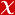## Discovery of new Delta Scuti Stars

Astrophysical Bulletin, vol.1, no.1, pp.1-10, 2022 (SCI-Expanded)• Publication Type: Article / Article
• Volume: 1 Issue: 1
• Publication Date: 2022
• Journal Name: Astrophysical Bulletin
• Journal Indexes: Science Citation Index Expanded (SCI-EXPANDED), Scopus
• Page Numbers: pp.1-10
• Çanakkale Onsekiz Mart University Affiliated: Yes

#### Abstract

Pulsating stars are remarkable objects for stellar astrophysics. Their pulsation frequencies allow us to probe the internal structure of stars. One of the most known groups of pulsating stars is $\delta$ Scuti variables which could be used to understand the energy transfer mechanism in A-F type stars. Therefore, in the current study, we focused on the discovery of $\delta$ Scuti stars. For this investigation, we followed some criteria. First, we inspected TESS database by eye and discovered some single stars that exhibit pulsation-like behaviour. Our second criterion is $T_{\rm eff}$ and $\log g$ range. The $\delta$ Scuti stars generally have $T_{\rm eff}$ and $\log g$ value in a range of 6300\,$-$\,8500\,K and 3.2\,$-$\,4.3, respectively. Hence, we selected the stars which have TIC $T_{\rm eff}$ and $\log g$ values in these ranges. The other criterion is the pulsating frequency. A frequency analysis was performed for all the candidate stars. In addition, $M_{V}$, $L$ and also $M_{bol}$ parameters of the target stars were determined to calculate the pulsation constants and show their positions in the H-R diagram. The final pulsation type classification was made by considering the frequency ranges and pulsation constants of the stars. As a result of the study, five $\delta$ Scuti, one $\gamma$ Doradus and four hybrid systems were discovered.